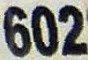## Friday, November 19, 2010

### 602

602 = 2 x 7 x 43.

602 is the maximum number of regions into which 25 circles divide the plane.

602 is the smallest number starting a block of five consecutive numbers with the same number of prime divisors (including multiplicity): 602 = 2 x 7 x 43; 603 = 3 x 3 x 67; 604 = 2 x 2 x 51; 605 = 5 x 11 x 11; 606 = 2 x 3 x 101.

602 are the first three digits of 5602.Telephone area code 602 covers most of the city of Phoenix, Arizona.